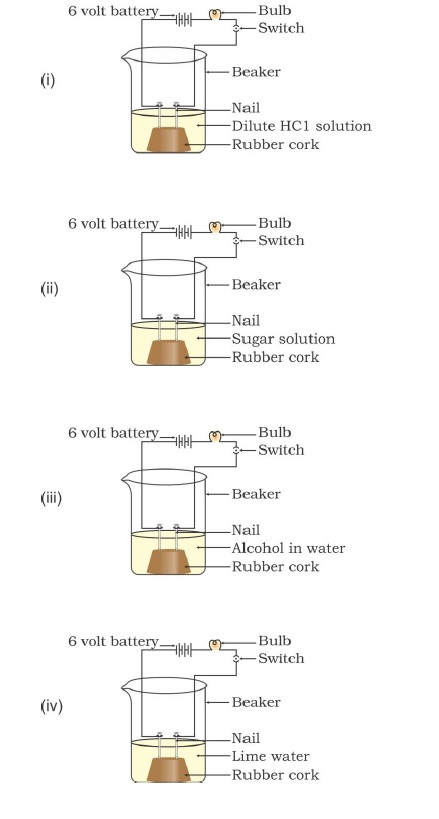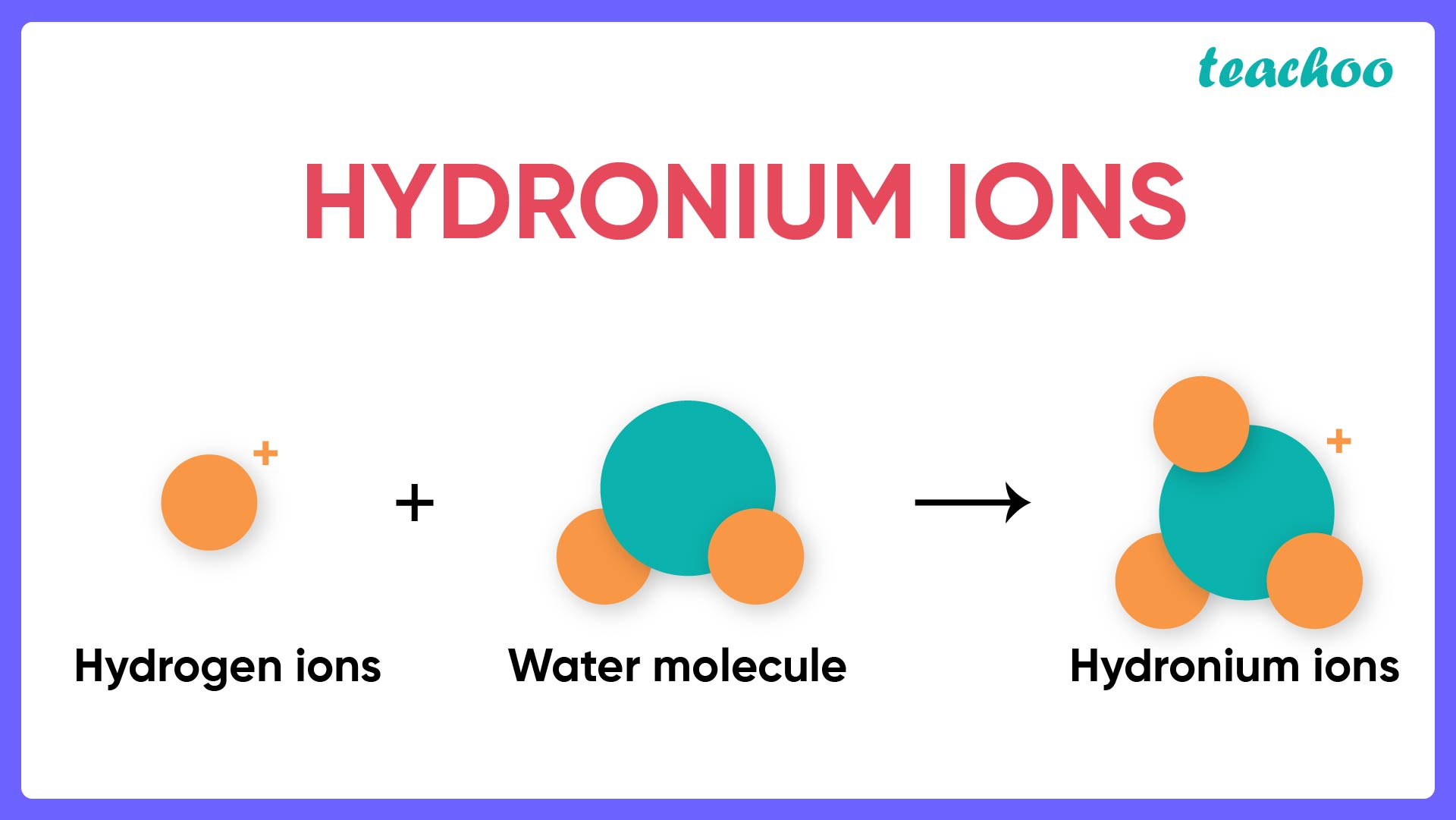Solutions - CBSE Class 10 Sample Paper for 2022 Boards - Science [MCQ]

Class 10
Solutions to CBSE Sample Paper - Science Class 10

## In which of the following setups would the bulb glow?## (D) i, ii and iv

Acids and Bases , when dissolved in water, conducts electricity … because it forms ions.From our options

1. For (i) - Since HCl is acidic, it forms ions in water. Thus, it conducts electricity.
2. For (ii) - Alcohol does not form ions in water. Thus, it does not conduct electricity.
3. For (ii) - Alcohol does not form ions in water. Thus, it does not conduct electricity.
4. For (iv) - Since Lime Water (Ca(OH) 2 ) is basic, it forms ions in water. Thus, it conducts electricity.

Thus, (i) and (iv) are correct

So, the correct answer is (b)